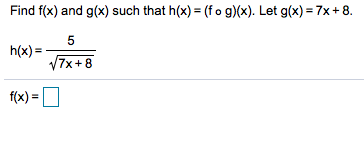# Find f(x) and g(x) such that hx) (f o g)(x). Let g(x) 7x + 8.5h(x)=7x+8fx)=

Question
2 views

Can you help me step by step with this problem?help_outlineImage TranscriptioncloseFind f(x) and g(x) such that hx) (f o g)(x). Let g(x) 7x + 8. 5 h(x)= 7x+8 fx)= fullscreen
check_circle

Step 1

To determine the function f(x) such that h(x) = (fog)(x).

Step 2

Given:

Step 3

The composition of the function f(x) and g(x) is denot...

### Want to see the full answer?

See Solution

#### Want to see this answer and more?

Solutions are written by subject experts who are available 24/7. Questions are typically answered within 1 hour.*

See Solution
*Response times may vary by subject and question.
Tagged in

### Calculus